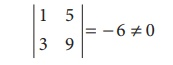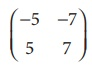Home | | Business Maths 12th Std | Rank of a Matrix: Concept

# Rank of a Matrix: Concept

With each matrix, we can associate a non-negative integer called its rank.

Rank of a Matrix

Matrices are one of the most commonly used tools in many fields such as Economics, Commerce and Industry.

We have already studied the basic properties of matrices. In this chapter we will study about the elementary transformations to develop new methods for various applications of matrices.

## Concept

With each matrix, we can associate a non-negative integer called its rank.

Definition 1.1

The rank of a matrix A is the order of the largest non-zero minor of A and is denoted by Žü(A)

In other words, A positive integer ŌĆśrŌĆÖ is said to be the rank of a non- zero matrix A, if

(i) there is atleast one minor of A of order ŌĆśrŌĆÖ which is not zero and

(ii) every minor of A of order greater than ŌĆśrŌĆÖ is zero.

Note

(i)   Žü(A)Ōēź 0

(ii) If A is a matrix of order m ├Ś n , then Žü(A)Ōēż minimum of {m,n}

(iii)The rank of a zero matrix is ŌĆś0ŌĆÖ

(iv) The rank of a non- singular matrix of order n ├Ś n is ŌĆśnŌĆÖ

Example 1.1

Find the rank of the matrixSolution:

Let A=Order of A is 2 ├Ś 2 Ōł┤          Žü(A)Ōēż 2

Consider the second order minorThere is a minor of order 2, which is not zero. Ōł┤Žü (A) = 2

Example 1.2

Find the rank of the matrixSolution:

Let A=Order of A is 2 ├Ś 2 Ōł┤Žü(A)Ōēż 2

Consider the second order minorSince the second order minor vanishes, Žü(A) ŌēĀ 2

Consider a first order minor |ŌłÆ5| ŌēĀ 0

There is a minor of order 1, which is not zero

Ōł┤ Žü (A) = 1

Example 1.3

Find the rank of the matrixSolution:

Let A=Order Of A is 3x3

Ōł┤ Žü (A) Ōēż 3

Consider the third order minor= 6 ŌēĀ 0

There is a minor of order 3, which is not zero

Ōł┤Žü (A) = 3.

Example 1.4

Find the rank of the matrixSolution:

Let A=Order Of A is 3x3

Ōł┤ Žü (A) Ōēż 3

Consider the third order minorSince the third order minor vanishes, therefore Žü(A) ŌēĀ 3

Consider a second order minorThere is a minor of order 2, which is not zero.

Ōł┤ Žü(A) = 2.

Example 1.5

Find the rank of the matrixSolution:

Let AOrder of A is 3 ├Ś 4

Ōł┤ Žü(A)Ōēż 3.

Consider the third order minorsSince all third order minors vanishes, Žü(A) ŌēĀ 3.

Now, let us consider the second order minors,

Consider one of the second order minorsThere is a minor of order 2 which is not zero.

Ōł┤Žü (A) = 2.

Study Material, Lecturing Notes, Assignment, Reference, Wiki description explanation, brief detail
12th Business Maths and Statistics : Chapter 1 : Applications of Matrices and Determinants : Rank of a Matrix: Concept |